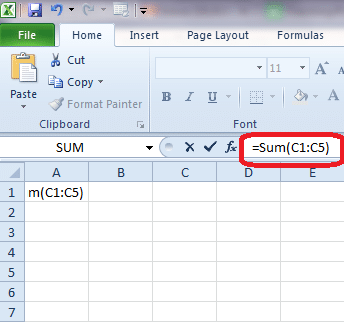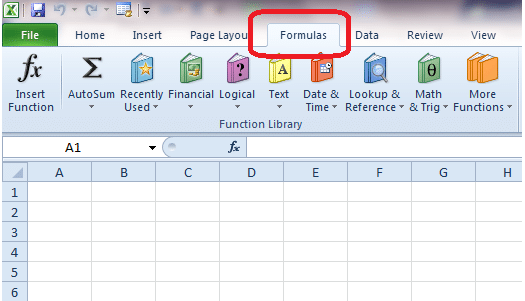Microsoft Excel has been the most popular spreadsheet software in the market for a while now.  Microsoft has added several features to its flagship software over the years and today Excel has everything you could ever need from a spreadsheet program. At the moment,Excel is used be a very large number of organizations across the world, so it’s important that you know how to handle the software to perform basic tasks. It can go on your resume as an additional skill and it will also give you a leg up at work. The software is very good at helping you perform basic tasks and automating calculations.We offer an introductory Excel course that you can take to get started with the basics of the software.

## Excel Functions

In this tutorial, we’re going to take a look at functions in Excel and how you can use them. Functions are an integral part of Excel. So what are Excel functions exactly? Microsoft maintains a large library of mathematical formula that can referenced to perform almost any mathematical task you can think of, from finding the average of a bunch of numbers to calculating the percentage profit your company is making this year. Excel has literally hundreds of functions stored in the library, which should be more than enough for your needs. However, in case you don’t find a function you’re looking for, you can create one yourself.

## The Basic Parts of a Function

A function has to be written in a certain way if you want to use it. A function in Excel looks something like this:

`=function_name (argument1, argument2)`

Here, the function_name will be the name of the function, which is usually easy to remember, like SUM. Once you begin to type the name in the function bar, a submenu will pop up that guides you through the syntax required to write the function. The argument parameter contains the data that your function will work on. A function can have multiple arguments (or bits of data) inside it. The comma is used to separate these arguments. Arguments must have parentheses “()” around them. Learn more about using Excel functions with this course.

## How to Write a Function

Let’s understand functions better with the help of a couple of examples. Take a look at the image below. Before you can begin using a function, you first need a space where you will obtain the result. You can just click on an empty cell anywhere in your spreadsheet and then begin writing the function. If at any time you need to modify the function, you can use the function bar at the top.Your result will now be obtained on the cell you clicked.In our example, we have clicked on cell A1. The function we have used is the SUM function. The SUM function calculates the sum of two or more numbers. These numbers can either be present in cells or you can specify them separately. Here, we have used the argument C1:C5. This tells excel to use the numbers found in cells C1 to C5 to calculate the sum. The colon “:” operator is used to specify the range in Excel.

In our example, we have left the cells blank. Why don’t you try filling out a few numbers in the cells C1 to C5 and see the result you get? To use the function, you just have to type it and hit enter. If you encounter a problem with any step, sign up for this course to see how it’s done. We guide you through the basics of the software, one step at a time, so that you’ll be able to identify mistakes and correct them quickly.

## Using the Function Library

You don’t need to remember all the functions in Excel. Frankly, they are too many and, once you know how the basics work, trying to learn them all isn’t required. To access these functions, you can click on the Formulas button on the menu at the top. A new submenu will open, which will contain formulae organized in various categories, like Recently, Financial, Date& Time and Math. In addition to these categories, you will find the AutoSum button, which contains the most used functions in Excel: like SUM, AVERAGE and COUNT.Using the functions in the library is easy. Assuming you have a bunch of numbers in your spreadsheet, all you have to do is click on an empty cell and click on the category of formulae you want. In the submenu, select the particular formula you are looking for. It will now automatically appear on the cell you clicked. Now, all you have to do is select the range of cells you want the result calculated for.

## The Insert Function Box

The formulas tab, shown in the image above, also has an insert function option. Click on it opens up an insert function box. This box is where you can search for functions you can’t seem to find, or you don’t know the category in which a particular function should belong to. You need to type a brief description of what the function you’re looking for is supposed to do, like “multiply two cells” or “compound interest”.

A number of formulae will now appear in a list below your query, and you can select a function that best matches your need. The formula, once you hit okay, will apply to the cell you have highlighted.

Now that you know the basics of how functions work and where you can locate them, your next step should be practicing the basic functions in Excel, like SUM and AVERAGE. You can take a look at some of our other Excel tutorials for help. Alternatively, you can just sign up for this Excel course and learn everything in one place, with minimum fuss.

Page Last Updated: June 2014